# Knowledge Base

## Solution 34598: Calculating Cubic Regressions on the TI-84 Plus C Silver Edition Graphing Calculator.

### How do I calculate a cubic regression on a TI-84 Plus C Silver Edition Graphing calculator?

Example: Find the cubic regression equation for the x and y values below.
xStat: {80, 84, 86, 91, 93, 96}
yStat: {10, 7, 9, 18, 19, 15}

To enter the data:
• Enter STAT edit mode by pressing [STAT] [ 1 ].
• Enter the data in the L1 and L2 lists, pressing [ENTER] after each entry.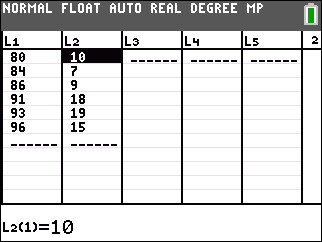• Press [2nd] [QUIT] to leave the editor.
• Verify that STAT WIZARDS is ON by pressing [MODE] and selecting ON next to STAT WIZARDS.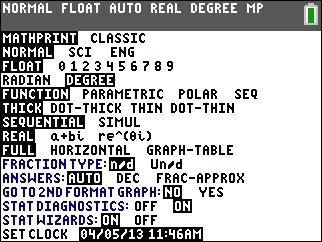To calculate the cubic Regression (ax3+bx2+cx+d):
• Enter the STAT mode again by pressing [STAT].
• Select CALC.
• Press  to select CubicReg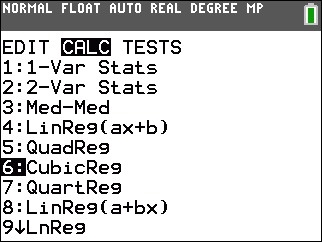• Specify which lists to use for the regression, press [2nd] [L1] for Xlist  and  [2nd] [ L2] for Ylist. ( The Xlist and Ylist should be populated by default)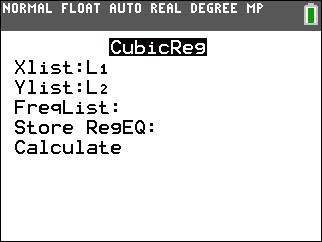• Press [ENTER] to perform the regression calculation.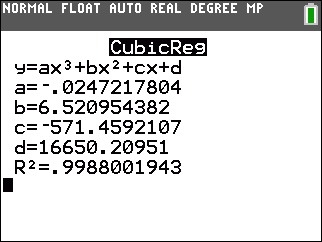The calculator will display the form of the equation (y=ax3+bx2+cx+d) and then list the values for the coefficients.

Please see the TI-84 Plus C Silver Edition guidebook for additional information.